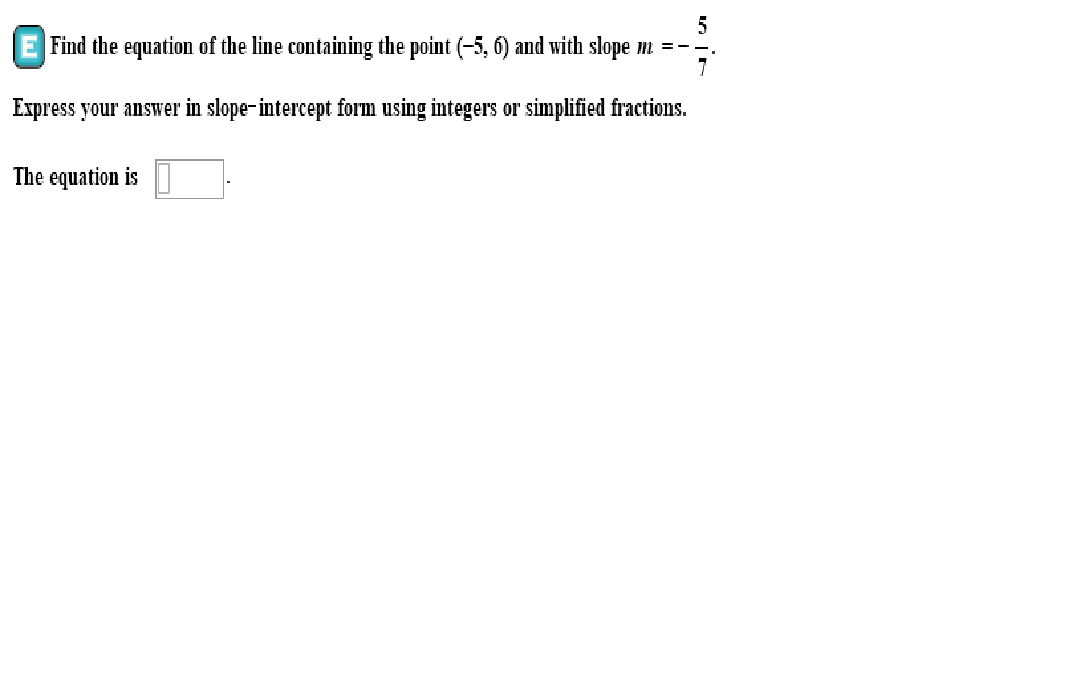# Find the equation of the line containing the point (-5, 6) and with slope m --Express your answer in slope-intercept form using integers or simplified fractions.The equation is

Question
103 viewshelp_outlineImage TranscriptioncloseFind the equation of the line containing the point (-5, 6) and with slope m -- Express your answer in slope-intercept form using integers or simplified fractions. The equation is fullscreen
check_circle

Step 1

The slope point form of a line equation with  slope m and passing through a point (x1,y1...

### Want to see the full answer?

See Solution

#### Want to see this answer and more?

Solutions are written by subject experts who are available 24/7. Questions are typically answered within 1 hour.*

See Solution
*Response times may vary by subject and question.
Tagged in

### Equations and In-equations